Thursday, October 22, 2020 |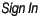Animal Query Calculators Articles Genetic Evaluation Breed Association Tools Data Services CDN Mobile Release Calendar 2019 2020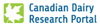Lifetime Performance Index (LPI) Formula 30-MAR-2020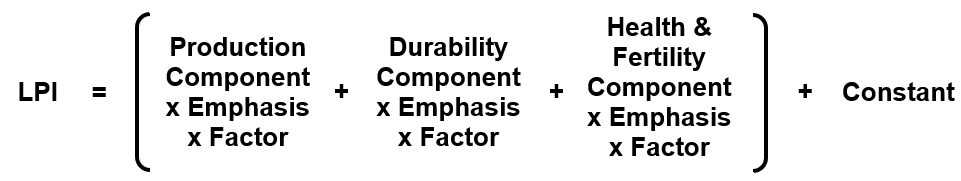Where the relative emphasis placed on each of the three main components in each breed is presented in the following table along with the multiplicative factors for each component.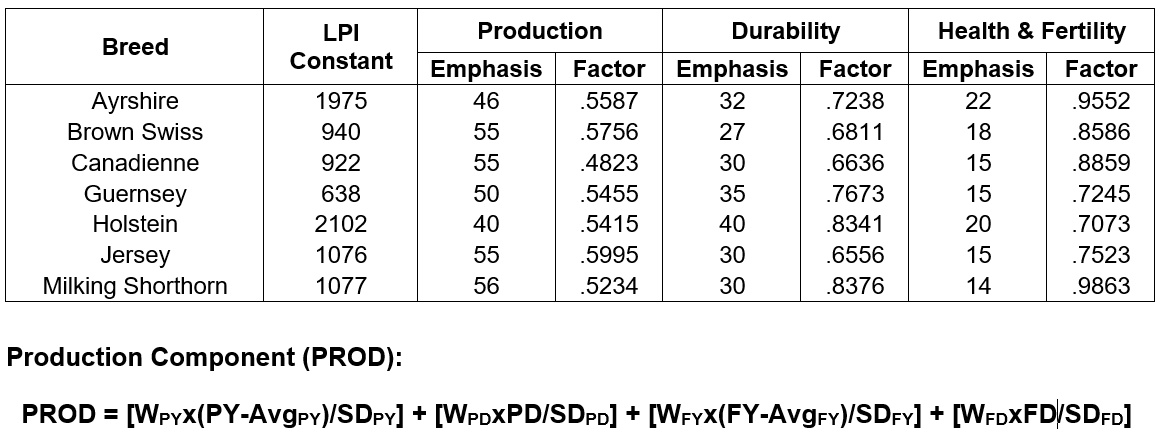Where PY = Protein Yield, PD = Protein Deviation, FY = Fat Yield and FD = Fat Deviation, which are standardized using the appropriate averages (Avg) and standard deviations (SD) and then multiplied by their respective relative weight (W), all of which are breed specific as outlined in the following table.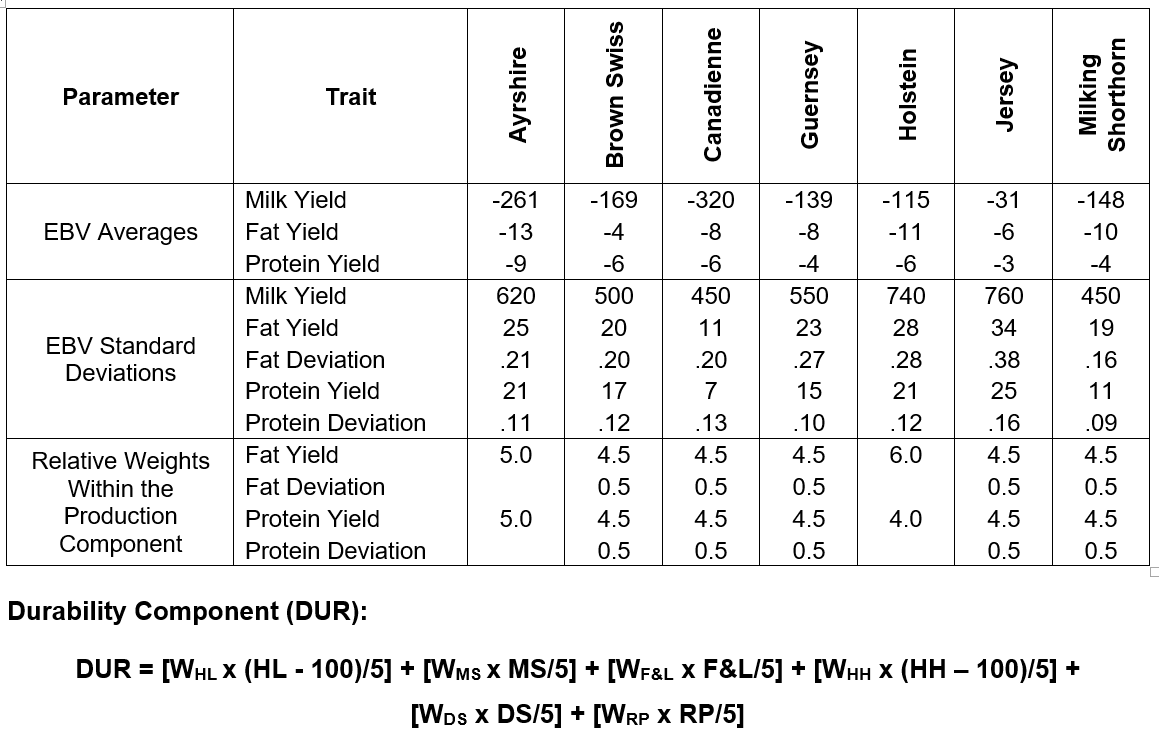Where HL = Herd Life, MS = Mammary System, F&L = Feet and Legs, HH = Hoof Health, DS = Dairy Strength and RP = Rump, and each trait is standardized using the appropriate averages and standard deviations and then multiplied by their respective relative weight (W) that is breed specific as outlined in the following table.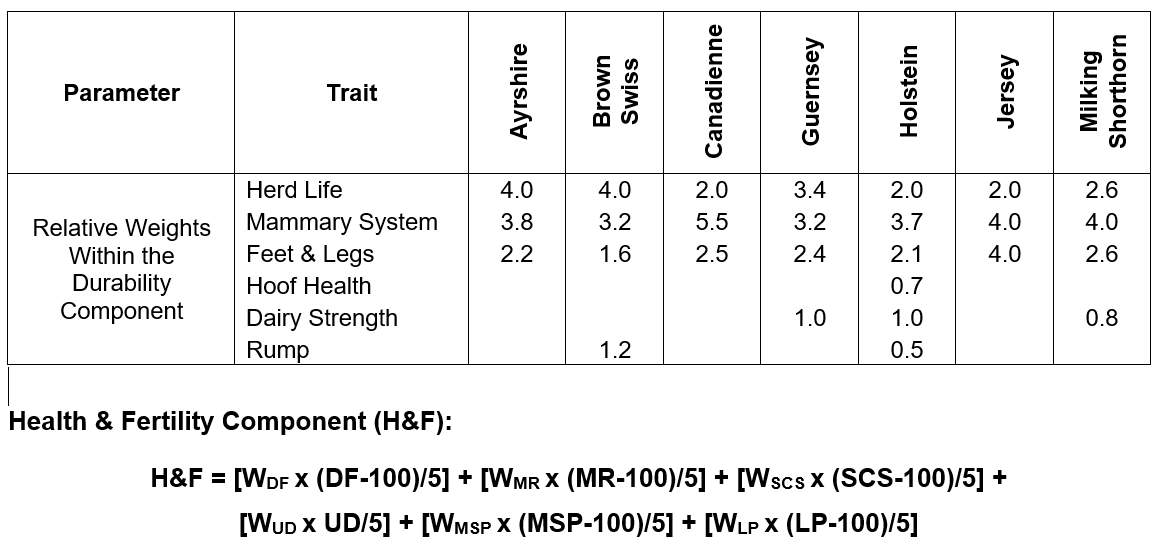Where DF = Daughter Fertility, MR = Mastitis Resistance, SCS = Somatic Cell Score, UD = Udder Depth, MSP = Milking Speed and LP = Lactation Persistency.  The relative weights for each trait (i.e.: WDF, WMR, WSCS, WUD, WMSP and WLP respectively), which are specific to each breed, are provided in the following table.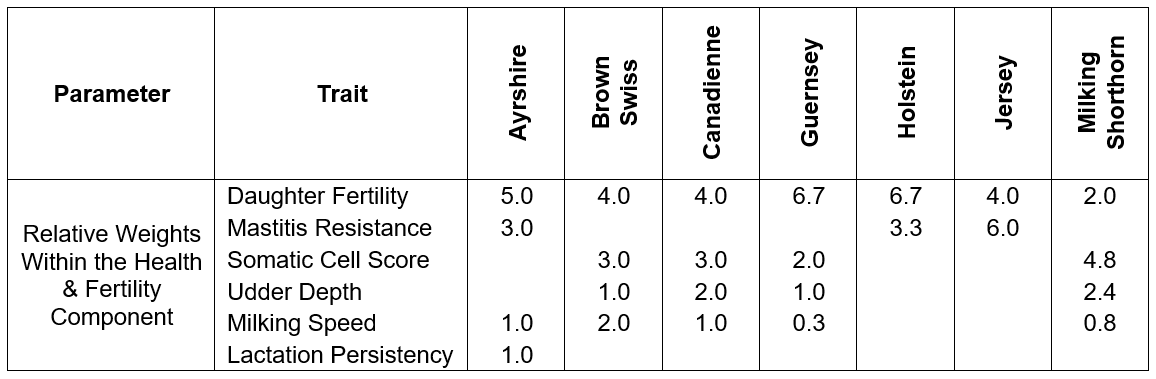Download a PDF copy of this article Previous LPI update documents: April 2019 April 2018 « back